Tuesday 29th September 2020CBSE Guess > Papers > Important Questions > Class XII > 2009 > Maths > Mathematics By Anil Kumar Tondak

CBSE CLASS XII

Application of Integrals

Q. 1. Find the area of the region in the first quadrant enclosed by the x-axis, the line y = x and the circle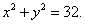Q. 2. Find the area of the region bounded by the ellipse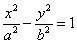.

Q. 3. Find the area of the region bounded by the parabola y = x2 and y =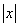.

Q. 4. Find the area of the smaller part of the circle x2 + y2 = a2 cut off by the linex=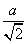.

Q. 5. Using integration, find the area of the region bounded by the triangle whose vertices are (1, 0), (2,2) and (3, 1).

Q. 6. Prove that the curves y2 = 4x and x2 = 4y divide the area of the square bounded by x=0, x=4, y=4 and y=0 into three equal parts.

Q. 7. Sketch the graph of y=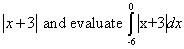Q. 8. Using the method of integration, find the area bounded by the curve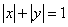.

Q. 9. Find the area of the smaller region bounded by the ellipse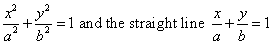.

Q. 10. Using integration, find the area of the triangular region, the equations of whose sides are y=2x + 1, y=3x +1 and  x = 4.

Q. 11. Find the area of the region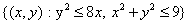Q. 12. Find the area of the region between the circles x2 + y2 = 4 and (x – 2)2 + y2 = 4.

Q. 13. Find the area bounded by the ellipse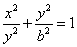and the co-ordinates x = ae and x = 0where b2=a2(1 – e2) and e<1.

Q. 14. Find the area bounded by the curve y2 = 4a2(x – 1) and the lines x = 1and y = 4a.

Q. 15. Using integration, find the area of the region bounded by the following curves, after making a rough sketch:
y = 1 +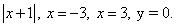Q. 16. Draw a rough sketch of the curves y = sinx and y = cosx as x varies from o to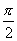and find the area of the region enclosed by them and x-axis.

Prepared By Anil Kumar Tondak
M.Sc.(Maths), M.Ed.,
M.A.(Eco),M.B.A.(Mkt)
Mob No. 9811363962

 Maths